# Slope of a Straight Line

The slope of a straight line is a number that tells you how steep it is. Another word for slope is gradient.

## Finding the Slope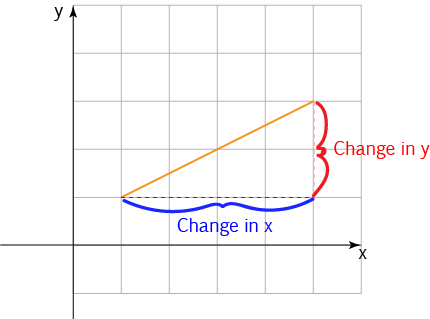The slope is given by the following formula:

$\text{Slope} = \dfrac{\text{Change in } y}{\text{Change in } x}$
So, we divide the change in the height of the line by the change in the horizontal distance it covers.

## Vertical Rise Over Horizontal Run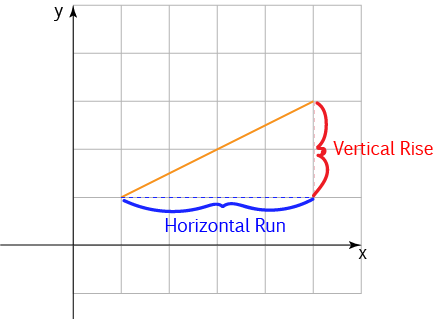We often call the change in height the vertical rise, and the change in horizontal distance the horizontal run, so the formula for the slope can be written as :

$\text{Slope} = \dfrac{\text{Vertical Rise}}{\text{Horizontal Run}}$
This gives exactly the same answer, it's just a change of terminology for the same things.

Let's calculate the slopes of some lines.

### Example 1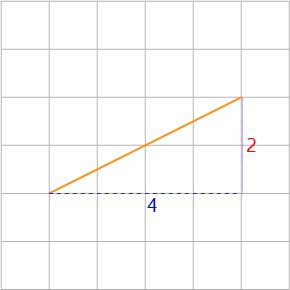The vertical rise is $2$ and the horizontal run is $4$, so the slope is

$\text{Slope} = \dfrac{2}{4} = \dfrac{1}{2}$

### Example 2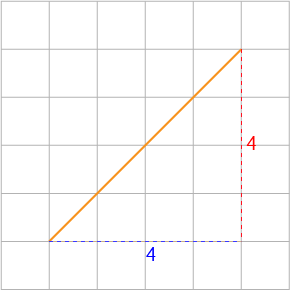The vertical rise is $4$ and the horizontal run is $4$, so the slope is

$\text{Slope} = \dfrac{4}{4} = 1$

Did you notice that this line is steeper than the line in Example 1? Its slope is larger, too.

### Example 3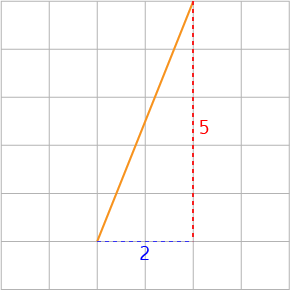The vertical rise is $5$ and the horizontal run is $2$, so the slope is

$\text{Slope} = \dfrac{5}{2} = 2.5$

This line is even steeper than the line in Example 2. Its slope is larger, too.

## Sign of the Slope

Slopes can be positive, negative or zero.

• When the slope is positive, the straight line is going uphill as we move from left to right. We say that the straight line function is increasing.
• When the slope is negative, the straight line is going downhill as we move from left to right. We say that the straight line function is decreasing.
• When the slope is zero, the straight line is horizontal. It slopes neither uphill nor downhill.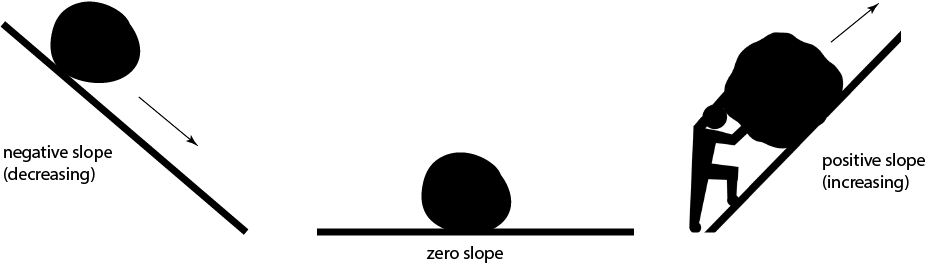In the picture, when the slope is negative, the boulder can roll down the hill. When the slope is zero, the boulder just sits on the flat. When the slope is positive, the man has to push the boulder up the hill.

### Example: Negative Slope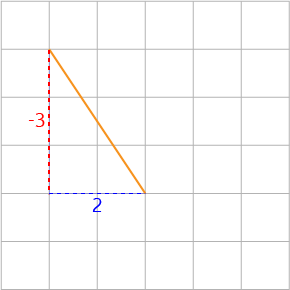The vertical rise is $-3$ as the line is sloping downwards, and the horizontal run is $2$, so the slope is

$\text{Slope} = \dfrac{-3}{2} = -1.5$

This line is sloping downhill, so it has a negative slope.

### Example: Zero Slope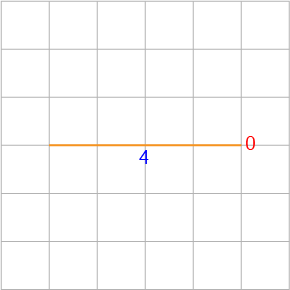The vertical rise is $0$ as the line is horizontal (flat), and the horizontal run is $4$, so the slope is

$\text{Slope} = \dfrac{0}{4} = 0$

This line is horizontal, so its slope is zero.

### Example: Vertical LineThe vertical rise is $4$, and the horizontal run is $0$ as the line is vertical, so the slope is

$\text{Slope} = \dfrac{4}{0} = ?$

That's a bit of a problem, isn't it? We say that the slope of vertical lines is undefined.

## Summary

The slope of a line that slopes uphill is positive.

The slope of a line that slopes downhill is negative.

The slope of a horizontal line is zero.

The slope of a vertical line is undefined.

Steeper (uphill) lines have larger slopes.

Steeper downhill lines have negative slopes that have larger sizes.

### Description

coordinate geometry is a branch of geometry where the position of the points on the plane is defined with the help of an ordered pair of numbers also known as coordinates. In this tutorial series, you will learn about vast range of topics such as Cartesian Coordinates, Midpoint of a Line Segment etc

### Audience

year 10 or higher, several chapters suitable for Year 8+ students.

### Learning Objectives

Explore topics related to Coordinates Geometry

Author: Subject Coach
You must be logged in as Student to ask a Question.